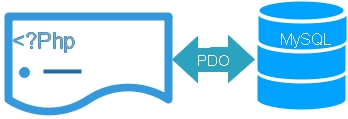# PDO inserting record to MySQL tableWe will learn how to insert or add record to a MySQL table by using PDO in PHP. Here we will only work on the database query and record insert part.

PHP PDO Parameterized query to insert data to MySQL table and getting lastInsertId() of the record

To keep the script simple and easy to understand we have omitted the data validation part. The table structure has unique constraints of userid so duplicate userid is not accepted. If you try to add same userid ( already exist) then you will get error message.

In your downloaded script there is a file pdo-insert.php . This file displays the form and after submission of the form data is posted to pdo-insert2.php file for storing in MySQL table.

Before using this page you must create the tables by using sql_dump file.

The detail of signup page with data validation is shown here. php_signup.php.

## collecting form data

``````\$userid=\$_POST['userid'];
\$name=\$_POST['name'];
\$status='T';//\$_POST['status'];``````

## LastInsertID

This gives you the value of the auto increment Id field. The value is created once a new record is added to the table. You can read more on this at mysql_insert_id() . WE assume that the PHP Pdo connection is already available. WE will directly go to query part.
``````\$status='T';// fixing status vlaue.
\$step=\$dbo->prepare(\$query);
\$step->bindParam(':userid',\$userid,PDO::PARAM_STR, 15);
\$step->bindParam(':name',\$name,PDO::PARAM_STR,25);
\$step->bindParam(':status',\$status,PDO::PARAM_STR);

if(\$step->execute()){
\$mem_id=\$dbo->lastInsertId();
echo " Thanks .. Your Membership id = \$mem_id ";
}
else{
}
``````

## Printing the error message.

The above code there is a if else condition checking, in case of failure of insert query it will display a message saying 'Not able to add data please contact Admin' however it does not tell about the error or mistake in the syntax. So to get more meaning full information from the database we have to use errorinfo() function. We will modify the part of the above code like this.
``````
if(\$step->execute()){
\$mem_id=\$dbo->lastInsertId();
echo " Thanks .. Your Membership id = \$mem_id ";
}
else{
print_r(\$step->errorInfo());
}
``````
Storing error message in a string and passing to front end
``````\$str='';
\$dbo->setAttribute(PDO::ATTR_EMULATE_PREPARES,false);
\$a=\$step->errorInfo();
while (list (\$key, \$val) = each (\$a)  ) {
\$str .= "<br>\$key -> \$val <br>";
}
``````

In the above code you can add current date like this ( only changes )
``````\$dt=date("Y-m-d");// Today's date
\$step->bindParam(':dt',\$dt,PDO::PARAM_STR,10);``````

## Changing date format to store in MySQL table

``````\$date = new DateTime(\$dt);
\$dt=\$date->format('Y-m-d');``````
Here \$dt is the variable storing date field value.

## To add null to a date field

If the date field is set to accept null data then here is the query to add
``````\$dt=null; // make it null
\$step->bindParam(':dt',\$dt,PDO::PARAM_STR,10);
``````
If you are receiving date field as blank from a form then use this PHP script.
``````if(strlen(\$dt) >2){
\$date = new DateTime(\$dt_approved);
\$dt=\$date->format('Y-m-d');
}else{
\$dt=null;
}
\$step=\$dbo->prepare("insert into table_name(dt) values(:dt)");
\$step->execute();``````
``Download Zip file to test your PHP PDO script``

Subscribe to our YouTube Channel here

## Subscribe

* indicates required
Subscribe to plus2netplus2net.com